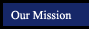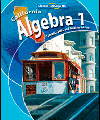Algebra 1 - Glencoe McGraw-Hill
Concepts, Skills and Problem SolvingChapter 1 The Language and Tools of Algebra Chapter 2 Solving Linear Equations Chapter 3 Functions and Patterns Chapter 4 Analyzing Linear Equations Chapter 5 Solving Linear Equations Chapter 6 Solving Linear Inequalities Chapter 7 Polynomials Chapter 8 Factoring Chapter 9 Quadratic and Exponential Functions Chapter 10 Radical Expressions and Triangles Chapter 11 Rational Expressions and Equations Chapter 12 Statistics and Probability